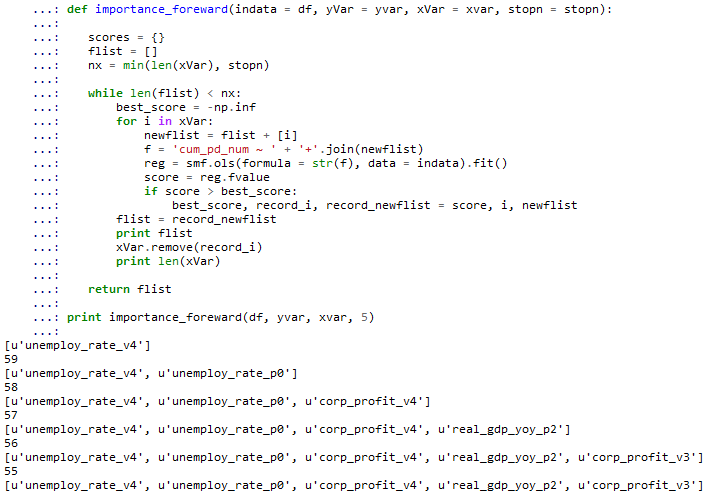# regression with forward variable selection

This is to mimic the selection = forward in SAS. The basic step is:

1. from all the independent vars, select the one having the highest F-value as the first input
2. for each var in the rest vars without the selected var, together with the selected var in step 1, run linear regression. and select the var with highest F-value
3. for the rest repeat as step 2, until reach the stop number or loop through all the variables.
import pandas as pd
import numpy as np
import matplotlib.pyplot as plt
import statsmodels.formula.api as smf

indata = df
xvar = df.columns.tolist()[12:]
yvar = 'cum_pd_num'
stopn = 5

'''
indata: is the data for analysis, in pd.DataFrame format
yvar: dependant variable name, it is string
xvar: list of independent variables name
stopn: number of variables to stop
'''

def importance_foreward(indata = df, yVar = yvar, xVar = xvar, stopn = stopn):

scores = {}
flist = []
nx = min(len(xVar), stopn)

while len(flist) < nx:
best_score = -np.inf
for i in xVar:
newflist = flist + [i]
f = 'cum_pd_num ~ ' + '+'.join(newflist)
reg = smf.ols(formula = str(f), data = indata).fit()
score = reg.fvalue
if score > best_score:
best_score, record_i, record_newflist = score, i, newflist
flist = record_newflist
print flist
xVar.remove(record_i)
print len(xVar)

return flist


the output is likewhich is the same as what SAS did.